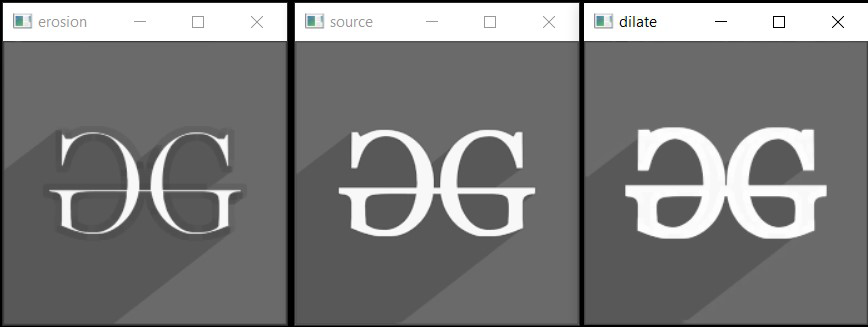Open in App
Not now

# Erosion and Dilation | Morphological Transformations in OpenCV in C++

• Last Updated : 22 Feb, 2021

Morphological Transformations are simple operations based on the shape of an image usually performed on a binary image. It takes our input image and a structuring element(kernel) which decides the nature of the operation.

In this article, we will discuss the two basic morphological filters erosion & dilation. Erosion erodes away the boundary and shrinks the size of the foreground object whereas Dilation dilates the boundary and hence increases the size of the foreground object. Before, moving into detail about them lets first understand what is a structuring element:

Structuring Element: A structuring element is a shape used to interact with a given image. It helps us to draw conclusions based on how it misses or fit in the image. It is used in morphological operations such as erosion, dilation, opening, closing, gradient, black-hat/top-hat transform. Open CV provides 3 shapes for kernel rectangular, cross, and elliptical.

Syntax:

getStructuringElement (shape, ksize, anchor)

Parameters: Below are the parameters required for the above syntax:

• shape: The shape of the structuring element could be any one of MORPH_RECT, MORPH_ELLIPSE, MORPH_CROSS.
• ksize: The size of the structuring element
• anchor: Anchor position inside the structuring element. The default value is [-1, -1} signifying position as the center of the structuring element.

Return Value: Structuring element of specified size and shape (Mat object).

### Erosion

Erosion is the morphological operation used to diminish the size of the foreground object. It is just like soil erosion and erodes away the boundary of the foreground object.

In this operation, the kernel is slid through the image and considers a pixel value 1 only when all the pixels in the structuring element have value 1. Otherwise, it is eroded. In this way, the pixels near the boundary will be discarded, and a shrunk foreground object inside the image is obtained.

Syntax:

erode (src, dst, kernel, anchor, iterations, borderType, borderValue)

Parameters:

• src: Input image
• dst: Output image
• kernel: The structuring element used for erosion.
• anchor: Anchor position inside the structuring element. The default value is [-1, -1} signifying position as the center of the structuring element.
• iterations: Number of times erosion is applied.
• borderType: Type of border (BORDER_CONSTANT, BORDER_REPLICATE, etc.)
• borderValue: Border value

Return Value: Output Image (Mat Object)

### Dilation

Dilation is the opposite of Erosion, instead of shrinking it expands the foreground object. In this operation structuring element(kernel) is slid through the image. But, here a pixel value 1 if at least one pixel has value 1. Hence, the object expands around the boundary and results in an expanded image.

Syntax:

dilate (src, dst, kernel, anchor, iterations, borderType, borderValue)

Parameters:

• src: Input image
• dst: Output image
• kernel: Structuring element used for dilation.
• anchor: Anchor position inside the structuring element. The default value is [-1, -1} signifying position as the center of the structuring element.
• iterations: Number of times dilation is applied.
• borderType: Type of border (BORDER_CONSTANT, BORDER_REPLICATE, etc.)
• borderValue: Border value

Return Value: Output Image (Mat Object)

Below is the C++ program to implement the above concepts:

## C++

 `// C++ program to implement the erosion``// and dilation``#include ``#include `` ` `// Library to include for drawing shapes``#include ``#include ``using` `namespace` `cv;``using` `namespace` `std;`` ` `// Driver Code``int` `main(``int` `argc, ``char``** argv)``{``    ``// Reading the Image``    ``Mat image = imread(``"C:/Users/harsh/Downloads/geeks.png"``,``                       ``IMREAD_GRAYSCALE);`` ` `    ``// Check if the image is created``    ``// successfully or not``    ``if` `(!image.data) {``        ``std::cout << ``"Could not open or find"``                  ``<< ``" the image\n"``;``        ``return` `0;``    ``}`` ` `    ``// Create a structuring element (SE)``    ``int` `morph_size = 2;``    ``Mat element = getStructuringElement(``        ``MORPH_RECT, Size(2 * morph_size + 1,``                         ``2 * morph_size + 1),``        ``Point(morph_size, morph_size));``    ``Mat erod, dill;`` ` `    ``// For Erosion``    ``erode(image, erod, element,``          ``Point(-1, -1), 1);`` ` `    ``// For Dilation``    ``dilate(image, dill, element,``           ``Point(-1, -1), 1);`` ` `    ``// Display the image``    ``imshow(``"source"``, image);``    ``imshow(``"erosion"``, erod);``    ``imshow(``"dilate"``, dill);``    ``waitKey();`` ` `    ``return` `0;``}`

Output Image:My Personal Notes arrow_drop_up